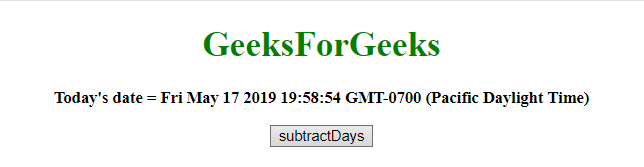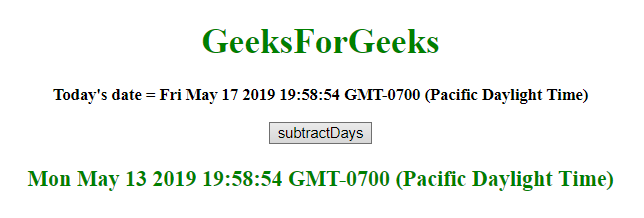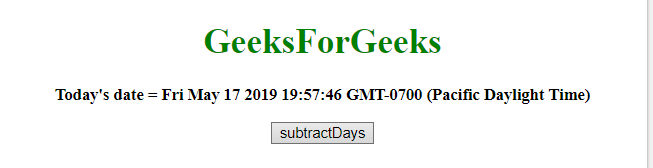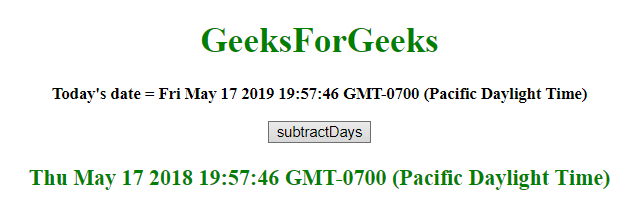Related Articles
Subtract days from Date object in JavaScript
• Last Updated : 24 May, 2019

Given a date and the task is to subtract days from date. To subtract days from date in JavaScript, some methods are used which are descibed velow:

• JavaScript getDate() Method: This method returns the day of the month (from 1 to 31) for the defined date.

Syntax:

```Date.getDate()
```

Return value: It returns a number from 1 to 31, representing the day of month.

• JavaScript setDate() Method: This method sets the day of month to date object.

Syntax:

```Date.setDate(day)
```

Parameters:

• day: It is required parameter. It specifies the integer representing the day of a month. Values expected values are 1-31, but other values are also allowed.

• 0 will result in the last day of the previous month.
• -1 will result in the day before the last day of the previous month.
• If the month has 31 days, 32 will result in the first day of the next month.
• If the month has 30 days, 32 will result in the second day of the next month.

Return value: It returns a number, denoting the number of milliseconds between the date object and midnight January 1 1970.

• JavaScript getTime() method: This method returns the number of milliseconds between midnight of January 1, 1970, and the specified date.

Syntax:

```Date.getTime()
```

Return value: It returns a number, representing the number of milliseconds since midnight January 1, 1970.

• JavaScript setTime() method: This method sets the date and time by adding/subtracting a defines number of milliseconds to/from midnight January 1, 1970.

Syntax:

```Date.setTime(millisec)
```

Parameters:

• millisec: It is required parameter. It specifies the number of milliseconds to be added/subtracted, midnight January 1, 1970.

Return value: It representing the number of milliseconds between the date object and midnight January 1 1970.

• Example 1: This example subtract 4 days from the var today by using setTime() and getTime() method.

 `  ` `<``html``>  ` `    ``<``head``>  ` `        ``<``title``>  ` `            ``Subtract days from Date object ` `        `` ` `    ``  ` `     `  `    ``<``body` `style` `= ``"text-align:center;"``>  ` `     `  `        ``<``h1` `style` `= ``"color:green;"` `>  ` `            ``GeeksForGeeks  ` `        `` ` `         `  `        ``<``p` `id` `= ``"GFG_UP"` `style` `= ``"font-size: 15px; font-weight: bold;"``> ` `        `` ` `         `  `        ``<``button` `onclick` `= ``"gfg_Run()"``>  ` `            ``subtractDays ` `        `` ` `         `  `        ``<``p` `id` `= ``"GFG_DOWN"` `style` `=  ` `            ``"color:green; font-size: 20px; font-weight: bold;"``> ` `        `` ` `         `  `        ``<``script``> ` `            ``var el_up = document.getElementById("GFG_UP"); ` `            ``var el_down = document.getElementById("GFG_DOWN"); ` `            ``var today = new Date(); ` `            ``el_up.innerHTML = "Today's date = " + today; ` `             `  `            ``Date.prototype.subtractDays = function(d) {  ` `                ``this.setTime(this.getTime() - (d*24*60*60*1000));  ` `                ``return this;  ` `            ``} ` `             `  `            ``function gfg_Run() { ` `                ``var a = new Date(); ` `                ``a.subtractDays(4); ` `                ``el_down.innerHTML = a; ` `            ``}          ` `        ``  ` `    ``  ` `                     `

Output:

• Before clicking on the button:• After clicking on the button:Example 2: This example subtract 365 days from the var today by using setDate() and getDate() method.

 `  ` `<``html``>  ` `    ``<``head``>  ` `        ``<``title``>  ` `            ``Subtract days from Date object ` `        `` ` `    ``  ` `     `  `    ``<``body` `style` `= ``"text-align:center;"``> ` `          `  `        ``<``h1` `style` `= ``"color:green;"` `>  ` `            ``GeeksForGeeks  ` `        `` ` `         `  `        ``<``p` `id` `= ``"GFG_UP"` `style` `= ``"font-size: 15px; font-weight: bold;"``> ` `        `` ` `         `  `        ``<``button` `onclick` `= ``"gfg_Run()"``>  ` `            ``subtractDays ` `        `` ` `         `  `        ``<``p` `id` `= ``"GFG_DOWN"` `style` `=  ` `            ``"color:green; font-size: 20px; font-weight: bold;"``> ` `        `` ` `         `  `        ``<``script``> ` `            ``var el_up = document.getElementById("GFG_UP"); ` `            ``var el_down = document.getElementById("GFG_DOWN"); ` `            ``var today = new Date(); ` `            ``el_up.innerHTML = "Today's date = " + today; ` `             `  `            ``Date.prototype.subtractDays= function(d) { ` `                ``this.setDate(this.getDate() - d); ` `                ``return this; ` `            ``} ` `             `  `            ``function gfg_Run() { ` `                ``var a = new Date(); ` `                ``a.subtractDays(365); ` `                ``el_down.innerHTML = a; ` `            ``}          ` `        ``  ` `    ``  ` `                     `

Output:

• Before clicking on the button:• After clicking on the button:My Personal Notes arrow_drop_up
Recommended Articles
Page :